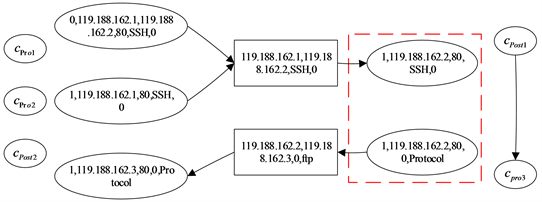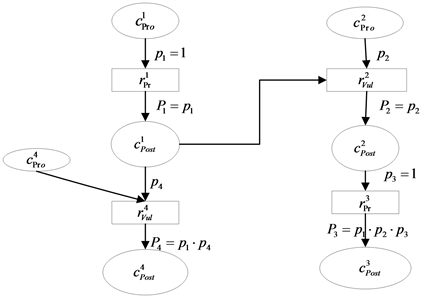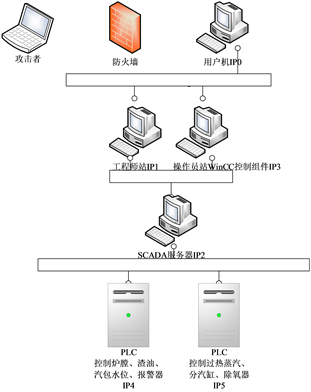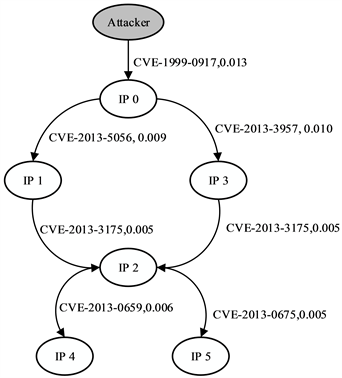# 基于属性攻击图的工控系统脆弱性量化方法A Method for Quantifying Vulnerability of Industrial Control System Based on Attribute Attack Graph

DOI: 10.12677/CSA.2021.112029, PDF, HTML, XML, 下载: 83  浏览: 146  科研立项经费支持

Abstract: A method for quantifying the vulnerability of industrial control system based on attack graph is proposed. First, the two dimensions of vulnerability existing in industrial control systems are analyzed, which are exploitation difficulty of vulnerability and vulnerability hazard. Some quantitative indexes of vulnerability are proposed by combining these dimensions with some concrete industrial aspects, such as defense strength, attack strength, physical loss, and information loss. Then, a specific grade division standard is formulated. By means of attack graph, the vulnerability of each attack path in industrial control system can be obtained by calculating each atomic attack expectation. Finally, a case of boiler control system is analyzed and simulated to verify the rationality of this method. Experimental results show that this method can analyzed the potential threats in industrial control systems more comprehensively and evaluate the vulnerability of each attack path more reasonably. The attack path that has the largest attack expectation can be obtained through simulation.

1. 引言

2. 属性攻击图模型

2.1. 属性攻击图模型定义

$\text{DT-AAG}=\left(C,R,E,p\right)$ (1)

1) 威胁转移条件属性集C

$C={C}_{Pro}\cup {C}_{Post}$ (2)

${C}_{Pro}=\left(ID,I{P}_{Pro},I{P}_{Post},Port,Vul,Pr\right)$ (3)

ID表示前置条件中攻击者的权限，且 $ID\in \left(0,1\right]$。当 $ID=0$ 时表示攻击者不具有该节点任何权限，当 $ID\in \left(0,1\right)$ 时表示攻击者具有该节点全部权限，当 $ID=1$ 时表示攻击者具有该节点全部权限； $I{P}_{Pro}$ 表示攻击的源IP； $I{P}_{Post}$ 表示攻击的目标IP；Port表示节点间连接的端口；Vul表示实施攻击的漏洞；Pr表示能够提升攻击者权限的服务访问关系，服务访问关系具体体现为协议。

${C}_{Post}=\left(I{D}^{\prime },I{P}^{\prime },Por{t}^{\prime },Vu{l}^{\prime },P{r}^{\prime }\right)$ (4)

$I{D}^{\prime }$ 表示攻击者实施攻击后获得的权限； $I{P}^{\prime }$ 表示获得权限节点的IP地址； $Por{t}^{\prime }$ 表示攻击利用的端口； $Vu{l}^{\prime }$ 表示实施攻击的漏洞； $P{r}^{\prime }$ 表示可提升权限的协议。

2) 威胁转移条件属性间的关系集R

$R=\left\{{r}_{Vul},{r}_{Pr}\right\}$ 是通过系统漏洞或协议关联主机或服务的关系节点集，其中 ${r}_{Vul}=\left(I{P}_{Pro},I{P}_{Post},Vul,0\right)$${r}_{Pr}=\left(I{P}_{Pro},I{P}_{Post},0,Pr\right)$ 分别表示漏洞节点和协议节点。

3) 连接条件属性和关系的边集E

$E=\left\{{C}_{Pro}\cdot R\right\}\cup \left\{R\cdot {C}_{Post}\right\}=\left\{{C}_{Pro}\cdot {r}_{Vul}\right\}\cup \left\{{r}_{Vul}\cdot {C}_{Post}\right\}\cup \left\{{C}_{Pro}\cdot {r}_{Pr}\right\}\cup \left\{{r}_{Pr}\cdot {C}_{Post}\right\}$ (5)

4) 威胁转移概率pFigure 1. DT-AAG attack diagram

2.2. 威胁转移概率p度量方法

1) 单步威胁转移概率度量

$ExpSco=20\cdot AV\cdot AC\cdot AU$ (6)

$ImpSco=10.41\cdot \left(1-\left(1-C\right)\cdot \left(1-I\right)\cdot \left(1-A\right)\right)$ (7)

$Risklevel=ExpSco+ImpSco$ (8)

$p=\lambda \cdot Risklevel$ (9)

2) 综合威胁转移概率度量

${P}_{k}={p}_{k}\cdot \underset{h=1}{\overset{i}{\prod }}{p}_{h}\underset{j=i+1}{\overset{k-1}{\prod }}{p}_{j}={p}_{k}\cdot \underset{h=1}{\overset{i}{\prod }}{p}_{h}$ (10)

${P}_{k}={p}_{k}\cdot \underset{h=1}{\overset{i}{\prod }}{p}_{h}$ (11)Figure 2. Multi-step attack threat transfer probability map

3. 脆弱性评估指标

$At{t}_{exp}=Vu{l}_{exp}×Vu{l}_{haz}$ (12)

3.1. 防御强度

1) 加密：工控系统中传输数据的方式主要有明文传输和密文传输，其中密文传输又包括AES (Advanced encryption standard)加密  和DES (Data encryption standard)加密 。加密的强度主要可以由密钥长度、破解难度和加减密时间来确定。

2) 认证：工控系统中的组件需要经过认证来鉴别数据的安全性，主要包括数字摘要、数字签名、数字信封和数字证书的四种认证方式，若在某个组件中部署的认证方式越多，则其越安全。其中数字锌粉由于采用双重加密技术来保证只有规定的接收者才能阅读数据，其安全性越高。

3) 信息屏障：主要的防护技术包括防火墙、入侵检测技术和访问控制。其中防火墙又可根据防御能力分为工业防火墙和商业防火墙；入侵检测技术的关键是如何从已知的数据中获得系统的正常行为或有关入侵行为的知识，可以分成模式匹配、神经网络、数据挖掘和数据融合；访问控制根据管理性质和安全级别又可分为基于授权规则的自主管理访问控制(Discretionary access control, DAC)、基于安全级的集中管理强制访问控制(Mandatory accesscontrol, MAC)和基于授权规则的集中管理角色访问控制(Role-based access control, RBAC)。

4) 物理屏障：主要指采取的物理防御手段，包括对外接口数量、组件所处位置、防静电、防火、防雷等。

3.2. 攻击强度

1) 攻击者数量：对某一漏洞利用的人越多，则脆弱性越高。本文参考NIST 7176标准 ，将攻击者数量分为三个等级：小于100、100~300和大于300。

2) 攻击者知识水平：经验丰富的攻击者显然比首次参与攻击的初学者具有更高的攻击成功概率，据此将知识水平按表1进行分级。Table 1. Knowledge of attackers

3) 威胁频率：参考《集散控制系统安全评估指南》中对威胁频率的赋值，如表2所示。

4) 漏洞危害性

5) 物理损失Table 3. Classification of component value

6) 信息损失

7) 等级划分标准打分Table 4. Classiﬁcation of three properties

4. 多指标归一与攻击图生成

4.1. 灰色关联度分析法

${x}_{i}=\left({x}_{i1},{x}_{x2},\cdots ,{x}_{ip}\right)$ (13)

1) 确定参考序列，在n个被评价对象中选出各项指标的最优值组成参考序列 ${x}_{0}$

${x}_{0}=\left({x}_{01},{x}_{02},\cdots ,{x}_{op}\right)$ (14)

2) 计算两集最大差 ${\Delta }_{\mathrm{max}}$ 和最小差 ${\Delta }_{\mathrm{min}}$。计算被评价对象序列与最优参考序列间的绝对差列 ${\Delta }_{ij}$

${\Delta }_{ij}=|{x}_{ij}-{x}_{0j}|$ (15)

${\Delta }_{\mathrm{max}}=\underset{1\le i\le n}{\mathrm{max}}\underset{1\le j\le p}{\mathrm{max}}\left({\Delta }_{ij}\right)$ (16)

${\Delta }_{\mathrm{min}}=\underset{1\le i\le n}{\mathrm{min}}\underset{1\le j\le p}{\mathrm{min}}\left({\Delta }_{ij}\right)$ (17)

3) 计算关联系数。计算第i个评价对象的第j个指标与最优参考序列间的关联系数 ${\delta }_{ij}$

${\delta }_{ij}=\frac{{\Delta }_{\mathrm{min}}+\rho {\Delta }_{\mathrm{max}}}{{\Delta }_{ij}+\rho {\Delta }_{\mathrm{max}}}$ (18)

4) 计算关联度。各评价对象与参考序列间的关联关系用关联度 ${\Upsilon }_{0i}$ 表示。

${\Upsilon }_{0i}=\frac{1}{p}\underset{k=1}{\overset{p}{\sum }}{\delta }_{ij}$$i=1,2,\cdots ,n$ (19)

${\Upsilon }_{0i}=\frac{1}{p}\underset{k=1}{\overset{p}{\sum }}{W}_{k}×{\delta }_{ij}$$i=1,2,\cdots ,n$ (20)

4.2. 攻击图生成算法

5. 案例分析

5.1. 漏洞利用难度量化

${x}_{0}=\left(3,4,2,4,3,2,3,2,3,4\right)$ (21)

${\Delta }_{\mathrm{max}}=3$

${\Delta }_{\mathrm{min}}=0$Figure 3. Topology of experimentTable 5. Information of component vulnerabilityTable 6. Values of VulexpTable 7. Degree of Vulexp for various vulnerabilities

5.2. 漏洞危害性量化Table 9. Degree of Vulhaz for various vulnerabilities

5.3. 攻击图生成Table 10. Attexp for various vulnerabilitiesFigure 4. Attack graphTable 11. Attexp for various paths

6. 总结

NOTES

*通讯作者。

  Chen, J., Wu, J., Liang, H., et al. (2020) Collaborative Trust Blockchain Based Unbiased Control Transfer Mechanism for Industrial Automation. IEEE Transactions on Industry Applications, 56, 4478-4488. https://doi.org/10.1109/TIA.2019.2959550  Humayed, A., Lin, J., Li, F., et al. (2017) Cyber-Physical Systems Security—A Survey. IEEE Internet of Things Journal, 4, 1802-1831. https://doi.org/10.1109/JIOT.2017.2703172  Desouza, K.C., Ahmad, A., Naseer, H., et al. (2020) Weaponiz-ing Information Systems for Political Disruption: The Actor, Lever, Effects, and Response Taxonomy (ALERT). Com-puters & Security, 88, Article ID: 101606. https://doi.org/10.1016/j.cose.2019.101606  刘芳. 信息系统安全评估理论及其关键技术研究[D]: [博士学位论文]. 长沙: 国防科学技术大学, 2005.  刘道远, 孙科达, 周君良, 等. 模糊综合评判法在电力企业网络信息安全评估中的应用[J]. 电信科学, 2020, 36(3): 38-45.  黄家辉, 冯冬芹, 王虹鉴. 基于攻击图的工控系统脆弱性量化方法[J]. 自动化学报, 2016, 42(5): 155-161.  Buldas, A., Gadyatskaya, O., Lenin, A., et al. (2020) Attribute Evaluation on Attack Trees with Incomplete Information. Computers & Security, 88, Article ID: 101630. https://doi.org/10.1016/j.cose.2019.101630  Lee, J., Moon, D., Kim, I., et al. (2019) A Semantic Approach to Improving Machine Readability of a Large-Scale Attack Graph. Journal of Supercomputing, 75, 3028-3045. https://doi.org/10.1007/s11227-018-2394-6  Islam, S.A. (2020) A Graph-Based Approach towards Hardware Trojan Vulnerability Analysis. Electronics Letters, 56, 868-871. https://doi.org/10.1049/el.2020.1005  杨英杰, 冷强, 潘瑞萱, 等. 基于属性攻击图的动态威胁跟踪与量化分析技术研究[J]. 电子与信息学报, 2019, 41(9): 2172-2179.  Lu, C., Feng, J., Chen, Y., et al. (2020) Tensor Robust Principal Component Analysis with a New Tensor Nuclear Norm. IEEE Transactions on Pattern Analysis and Machine Intelligence, 42, 925-938. https://doi.org/10.1109/TPAMI.2019.2891760  Wen, C., Huang, X. and Shen, C. (2020) Multiple-Pass En-hanced Raman Spectroscopy for Fast Industrial Trace Gas Detection and Process Control. Journal of Raman Spectros-copy, 51, 781-787. https://doi.org/10.1002/jrs.5838  杨英杰, 冷强, 常德显, 等. 基于属性攻击图的网络动态威胁分析技术研究[J]. 电子与信息学报, 2019, 41(8): 1838-1846.  Cao, J., et al. (2021) Hy-brid-Triggered-Based Security Controller Design for Networked Control System under Multiple Cyber Attacks. Infor-mation Sciences, 548, 69-84. https://doi.org/10.1016/j.ins.2020.09.046  Sheikhpour, S., Mahani, A. and Bagheri, N. (2019) Practical Fault Resilient Hardware Implementations of Advanced Encryption Standard. IET Circuits, Devices & Systems, 13, 596-606. https://doi.org/10.1049/iet-cds.2018.5235  He, D., Liu, X., Zheng, J., et al. (2020) A Lightweight and Intelligent Intrusion Detection System for Integrated Electronic Systems. IEEE Net-work.  Yu, B., Cai, Y. and Wu, D. (2020) Joint Access Control and Resource Allocation for Short-Packet-Based mMTC in Status Update Systems. IEEE Journal on Selected Areas in Communications. https://doi.org/10.1109/JSAC.2020.3018801  Figueroa-Lorenzo, S., Añorga, J. and Arrizabalaga, S. (2020) A Survey of IIoT Protocols: A Measure of Vulnerability Risk Analysis Based on CVSS. ACM Computing Surveys, 53, 1-53. https://doi.org/10.1145/3381038  Mehlawat, M.K., Gupta, P. and Mahajan, D. (2020) A Multi-Period Multi-Objective Optimization Framework for Software Enhancement and Component Evaluation, Selection and Integra-tion. Information Sciences, 523, 91-110. https://doi.org/10.1016/j.ins.2020.02.076  Ikram, M., Sroufe, R., Rehman, E., et al. (2020) Do Quality, Envi-ronmental, and Social (QES) Certifications Improve International Trade? A Comparative Grey Relation Analysis of De-veloping vs. Developed Countries. Physica A: Statistical Mechanics and Its Applications, 545, Article ID: 123486. https://doi.org/10.1016/j.physa.2019.123486  Gui, C.-Y., Zheng, L. and He, B.S. (2019) A Survey on Graph Processing Accelerators: Challenges and Opportunities. Journal of Computer Science and Technology, 34, 339-371. https://doi.org/10.1007/s11390-019-1914-z  Reynoso-Meza, G., et al. (2016) Preference Driven Mul-ti-Objective Optimization Design Procedure for Industrial Controller Tuning. Information Sciences: An International Journal, 339, 108-131. https://doi.org/10.1016/j.ins.2015.12.002